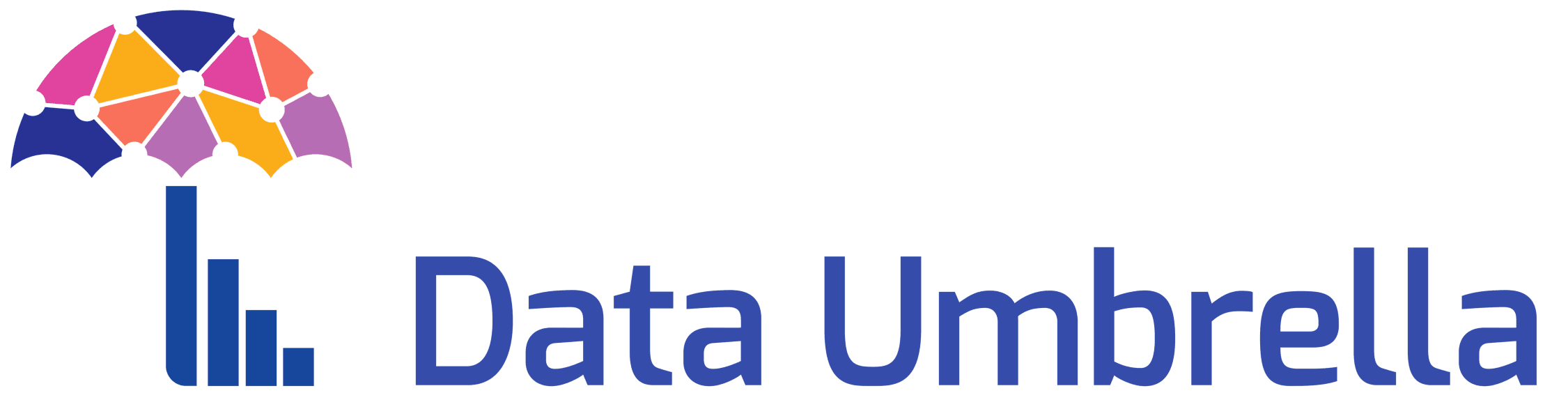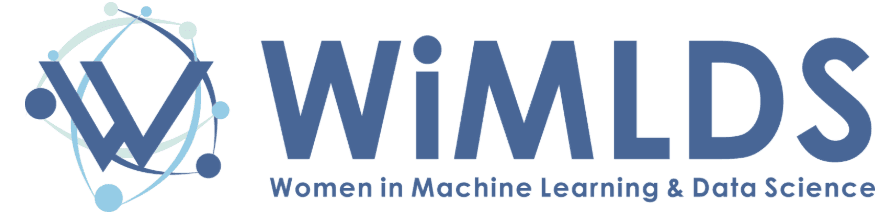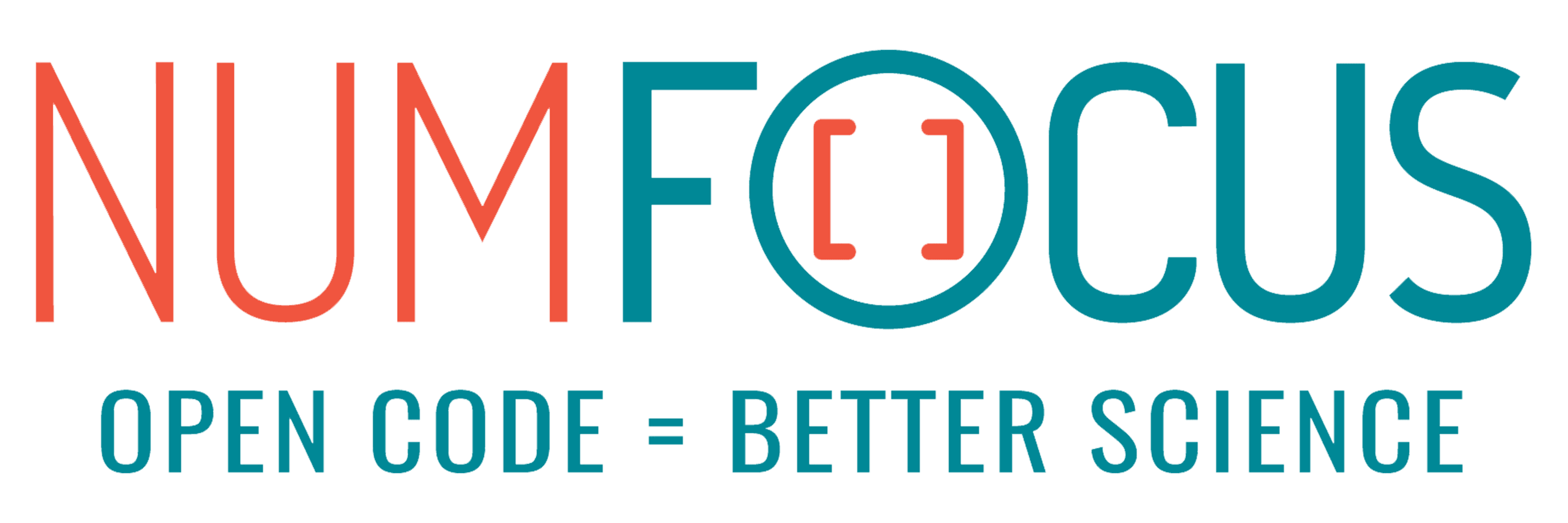# InferOpt.jl: combinatorial optimization in ML pipelines

07/29/2022, 5:00 PM5:30 PM UTC
Purple

#### Abstract:

We present InferOpt.jl, a generic package for combining combinatorial optimization algorithms with machine learning models. It has two purposes:

• Increasing the expressivity of learning models thanks to new types of structured layers.
• Increasing the efficiency of optimization algorithms thanks to an additional inference step.

Our library provides wrappers for several state-of-the-art methods in order to make them compatible with Julia's automatic differentiation ecosystem.

### Overview

We focus on a generic prediction problem: given an instance `x`, we want to predict an output `y` that minimizes the cost function `c(y)` on a feasible set `Y(x)`. When `Y(x)` is combinatorially large, a common approach in the literature is to exploit a surrogate optimization problem, which is usually a Linear Program (LP) `max_y θᵀy`.

A typical use of InferOpt.jl is integrating the optimization problem (LP) into a structured learning pipeline of the form `x -> θ -> y`, where the cost vector `θ = φ_w(x)` is given by an ML encoder. Our goal is to learn the weights `w` in a principled way. To do so, we consider two distinct paradigms:

1. Learning by experience, whereby we want to minimize the cost induced by our pipeline using only past instances `x`.
2. Learning by imitation, for which we have "true" solutions `y` or cost vectors `θ` associated with each past instance `x`.

We provide a unified framework to derive well-known loss functions, and we pave the way for new ones. Our package will be open-sourced in time for JuliaCon 2022.

### Related works

InferOpt.jl gathers many previous approaches to derive (sub-)differentiable layers in structured learning:

In addition, we provide several tools for directly minimizing the cost function using smooth approximations.

### Package content

Since we want our package to be as generic as possible, we do not make any assumption on the kind of algorithm used to solve combinatorial problems. We only ask the user to provide a callable `maximizer`, which takes the cost vector `θ` as argument and returns a solution `y`: regardless of the implementation, our wrappers can turn it into a differentiable layer.

As such, our approach is different from that of DiffOpt.jl, in which the optimizer has to be a convex JuMP.jl model. It is also different from ImplicitDifferentiation.jl, which implements a single approach for computing derivatives (whereas we provide several), and does not include structured loss function.

All of our wrappers come with their own forward and reverse differentiation rules, defined using ChainRules.jl. As a result, they are compatible with a wide range of automatic differentiation backends and machine learning libraries. For instance, if the encoder `φ_w` is a Flux.jl model, then the wrapped optimizer can also be included as a layer in a `Flux.Chain`.

### Examples

We include various examples and tutorials to apply this generic framework on concrete problems. Since our wrappers are model- and optimizer-agnostic, we can accommodate a great variety of algorithms for both aspects.

On the optimization side, our examples make use of:

• Mixed-Integer Linear Programs;
• Shortest path algorithms;
• Scheduling algorithms;
• Dynamic Programming.

On the model side, we exploit the following classes of predictors:

• Generalized Linear Models;
• Convolutional Neural Networks;
• Graph Neural Networks.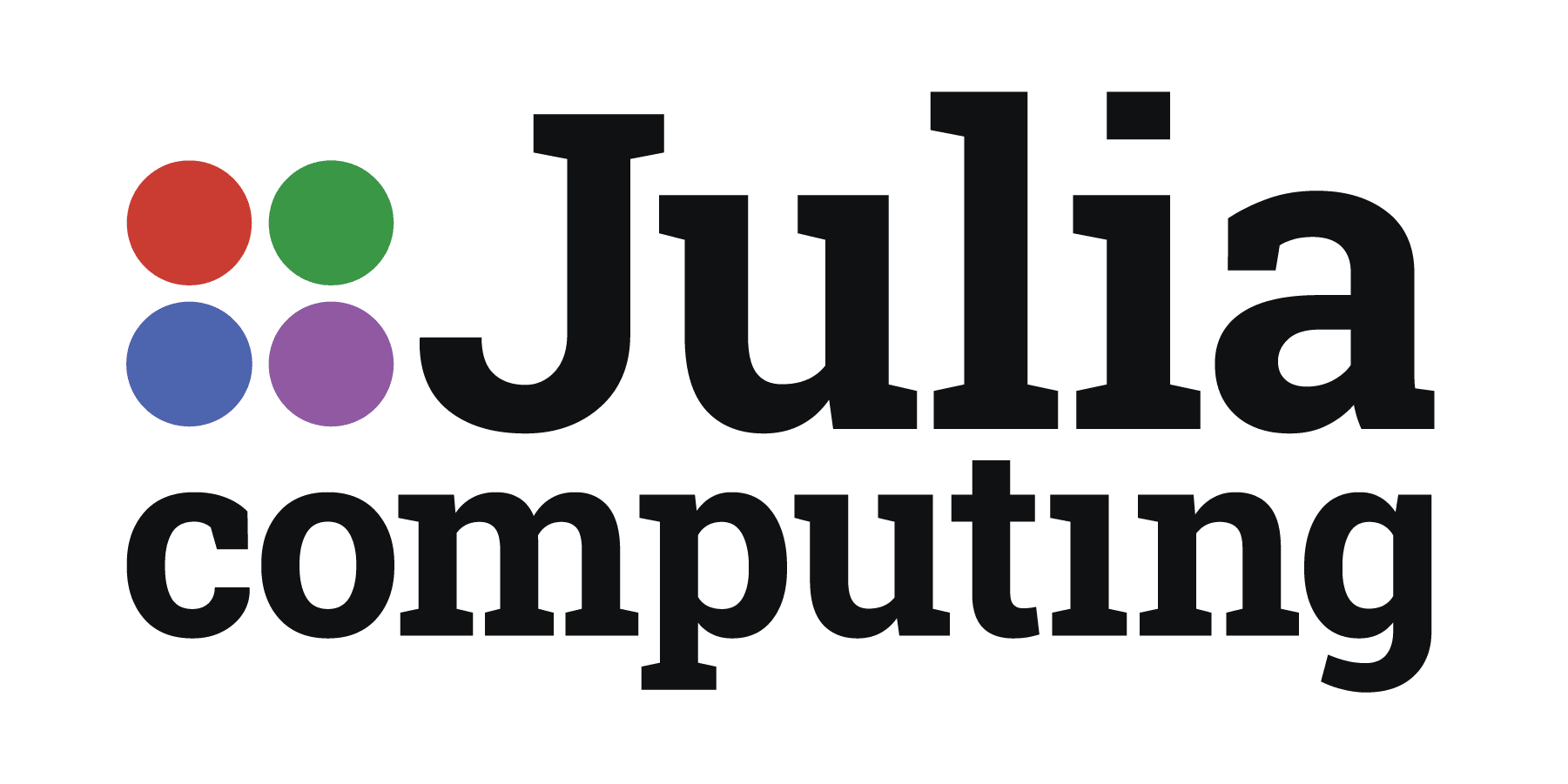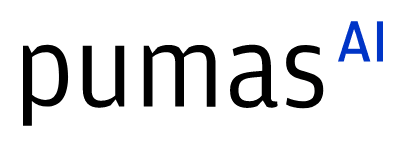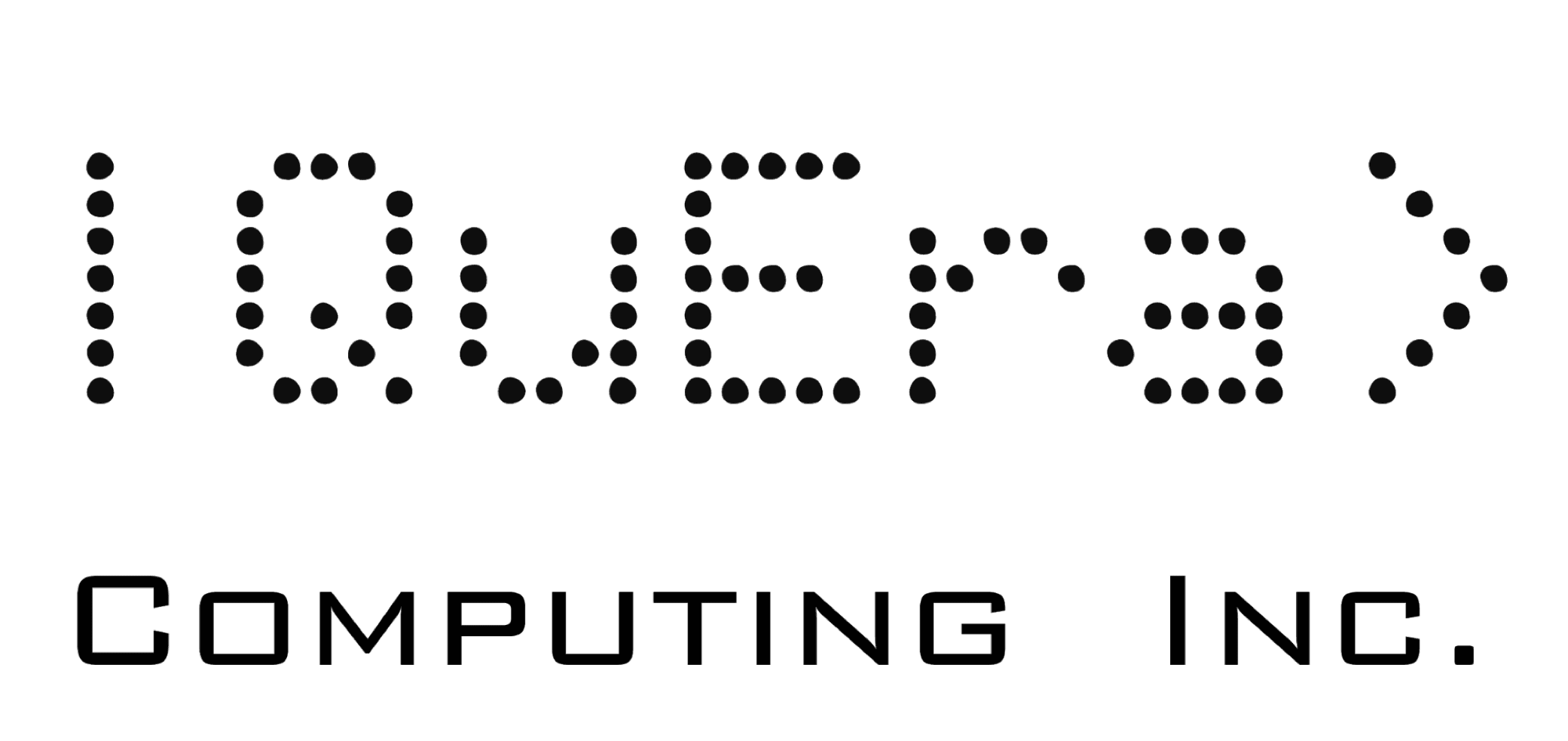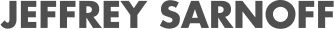## Media partners## Community partners